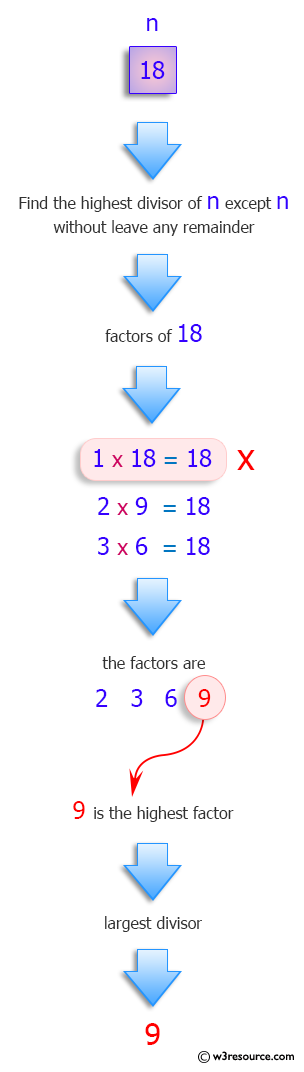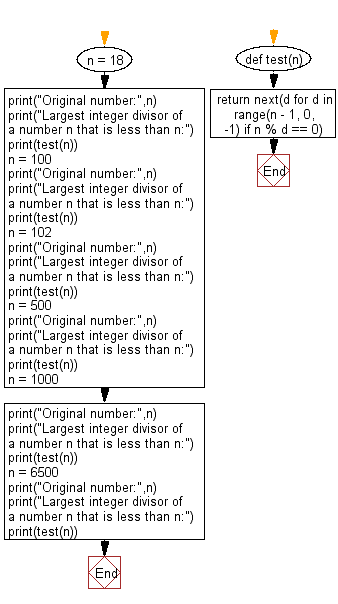﻿ Python: Find the largest integer divisor of a number n that is less than n - w3resource# Python: Find the largest integer divisor of a number n that is less than n

## Python Programming Puzzles: Exercise-37 with Solution

A divisor is a number that divides another number either completely or with a remainder.

Write a Python program to find the largest integer divisor of a number n that is less than n.

```Input:
18
Output:
9
Input:
100
Output:
50
Input:
102
Output:
51
Input:
500
Output:
250
Input:
1000
Output:
500
Input:
6500
Output:
3250
```

Pictorial Presentation:Sample Solution:

Python Code:

``````#License: https://bit.ly/3oLErEI

def test(n):
return next(d for d in range(n - 1, 0, -1) if n % d == 0)

n = 18
print("Original number:",n)
print("Largest integer divisor of a number n that is less than n:")
print(test(n))
n = 100
print("Original number:",n)
print("Largest integer divisor of a number n that is less than n:")
print(test(n))
n = 102
print("Original number:",n)
print("Largest integer divisor of a number n that is less than n:")
print(test(n))
n = 500
print("Original number:",n)
print("Largest integer divisor of a number n that is less than n:")
print(test(n))
n = 1000
print("Original number:",n)
print("Largest integer divisor of a number n that is less than n:")
print(test(n))
n = 6500
print("Original number:",n)
print("Largest integer divisor of a number n that is less than n:")
print(test(n))
``````

Sample Output:

```Original number: 18
Largest integer divisor of a number n that is less than n:
9
Original number: 100
Largest integer divisor of a number n that is less than n:
50
Original number: 102
Largest integer divisor of a number n that is less than n:
51
Original number: 500
Largest integer divisor of a number n that is less than n:
250
Original number: 1000
Largest integer divisor of a number n that is less than n:
500
Original number: 6500
Largest integer divisor of a number n that is less than n:
3250
```

Flowchart:## Visualize Python code execution:

The following tool visualize what the computer is doing step-by-step as it executes the said program:

Python Code Editor :

Have another way to solve this solution? Contribute your code (and comments) through Disqus.

What is the difficulty level of this exercise?

Test your Programming skills with w3resource's quiz.

﻿

## Python: Tips of the Day

Clamps num within the inclusive range specified by the boundary values x and y:

Example:

```def tips_clamp_num(num,x,y):
return max(min(num, max(x, y)), min(x, y))
print(tips_clamp_num(2, 4, 6))
print(tips_clamp_num(1, -1, -6))
```

Output:

```4
-1
```